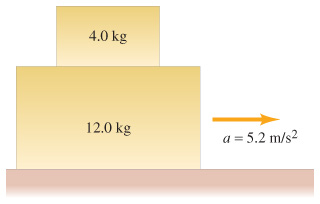# Problem: A 4.0-kg block is stacked on top of a 12.0-kg block, which is accelerating along a horizontal table at a = 5.2 m/s2. Let μk = μs = μ.(a) What minimum μ between the two blocks will prevent the 4.0-kg{ m kg}  block from sliding off?(b) If μ is only half this value, what is the acceleration of the 4.0-kg{ m kg}  block with respect to the table?(c) Repeat B with respect to the 12.0-kg block.(d) What force must be applied to the 12.0-kg block in A, assuming the table is frictionless?(e) What force must be applied to the 12.0-kg block in B, assuming the table is frictionless?

###### FREE Expert Solution
83% (232 ratings)
###### Problem Details

A 4.0-kg block is stacked on top of a 12.0-kg block, which is accelerating along a horizontal table at a = 5.2 m/s2. Let μk = μs = μ.
(a) What minimum μ between the two blocks will prevent the 4.0-kg  block from sliding off?
(b) If μ is only half this value, what is the acceleration of the 4.0-kg  block with respect to the table?
(c) Repeat B with respect to the 12.0-kg block.
(d) What force must be applied to the 12.0-kg block in A, assuming the table is frictionless?
(e) What force must be applied to the 12.0-kg block in B, assuming the table is frictionless?